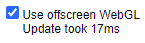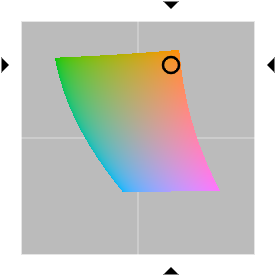## Wednesday, 5 May 2021

### Colour Pick 3: WebGL

If you look in the bottom-left corner of the Colour Pick experiment web page, you'll see a checkbox:Colour Pick WebGL checkbox

This is an optimization. It turns out that updating even a few canvases interactively whilst the user is dragging the mouse can produce very "laggy" responses. The problem is that some of the canvases draw non-trivial shapes with each pixel's colour computed on-the-fly. For example:CIE L*a*b* gamut canvas

The canonical way of updating a single pixel in a canvas via JavaScript is something akin to this:

var context = canvas.getContext("2d");
context.fillStyle = rgb;
context.fillRect(x, y, 1, 1);

This is fine for a few pixels but doesn't scale well. WebGL fragment shaders, on the other hand, are ideal for updating rectangular regions like this on a pixel-by-pixel basis. However, you cannot use both "2d" and "webgl" contexts on the same canvas. Consequently, the JS script, uses an off-screen "webgl" canvas to utilise fragment shaders and then "blits" the result to the on-screen canvas.

WebGL fragment shaders are only guaranteed to conform to OpenGL Shader Language (GLSL) version 1.00, so you have to be a bit conservative with your shader programming sometimes.

The "FRAGMENT_SHADER_SOURCE" variable at the top of the colourpick.js script contains the interesting GLSL code to perform the various colour space conversions.

The first function converts an HSL/HSV-style hue in the range zero to one into a fully-saturated RGB triple:

vec3 hue2rgb(float h) { float r = abs(h * 6.0 - 3.0) - 1.0; float g = 2.0 - abs(h * 6.0 - 2.0); float b = 2.0 - abs(h * 6.0 - 4.0); return clamp(vec3(r, g, b), 0.0, 1.0); }

The next function converts a red/green/blue triple to hue/chroma/value based on the work by

vec3 rgb2hcv(vec3 rgb) { vec4 p = (rgb.g < rgb.b) ? vec4(rgb.bg,-1.0,2.0/3.0) : vec4(rgb.gb,0.0,-1.0/3.0); vec4 q = (rgb.r < p.x) ? vec4(p.xyw, rgb.r) : vec4(rgb.r, p.yzx); float c = q.x - min(q.w, q.y); float h = abs((q.w - q.y) / (6.0 * c + 1.0e-10) + q.z); return vec3(h, c, q.x); }

Next we have our first true colour space conversion: HSV to RGB:

vec3 hsv2rgb(vec3 hsv) { vec3 rgb = hue2rgb(hsv.x); return ((rgb - 1.0) * hsv.y + 1.0) * hsv.z; }

HSL to RGB is similar:

vec3 hsl2rgb(vec3 hsl) { vec3 rgb = hue2rgb(hsl.x); return (rgb - 0.5) * (1.0 - abs(2.0 * hsl.z - 1.0)) * hsl.y + hsl.z; }

HWB (hue/whiteness/blackness) to RGB is also similar:

vec3 hwb2rgb(vec3 hwb) { vec3 rgb = hue2rgb(hwb.x); return rgb * (1.0 - hwb.y - hwb.z) + hwb.y; }

Converting "linear" RGB to sRGB requires component-wise conditionals. I'd love to be able to use 'vec3 mix(vec3, vec3, bvec3)' here, but basic WebGL doesn't support it, so we have to make sure that both "branches" of the conditional are finite:

vec3 lrgb2srgb(vec3 rgb) { vec3 linear = rgb * 12.92; vec3 nonlinear = 1.055 * pow(max(rgb, 0.0), vec3(1.0 / 2.4)) - 0.055; return mix(nonlinear, linear, vec3(lessThan(linear, vec3(0.04045)))); }

Converting CIE XYZ to linear RGB is a simple matrix multiplication, but basic WebGL doesn't support constant matrix initialization:

vec3 xyz2lrgb(vec3 xyz) { return vec3(dot(xyz, vec3(+3.2404542, -1.5371385, -0.4985314)), dot(xyz, vec3(-0.9692660, +1.8760108, +0.0415560)), dot(xyz, vec3(+0.0556434, -0.2040259, +1.0572252))); }

We have to avoid division by zero when converting CIE xyY to CIE XYZ:

vec3 xyy2xyz(vec3 xyy) { float d = xyy.z / max(xyy.y, 1.0e-10); return vec3(xyy.x * d, xyy.z, (1.0 - xyy.x - xyy.y) * d); }

We assume a D65 illuminant and a 2-degree standard colormetric observer for converting CIE L*a*b* to CIE XYZ. Again, it would be nice to use 'vec3 mix(vec3, vec3, bvec3)' in the final statement, but basic WebGL doesn't support it:

vec3 lab2xyz(vec3 lab) { float fy = (lab.x + 16.0) / 116.0; float fx = fy + lab.y * 0.002; float fz = fy - lab.z * 0.005; vec3 fxyz = vec3(fx, fy, fz); vec3 linear = (fxyz * 3132.0 - 432.0) / 24389.0; vec3 nonlinear = fxyz * fxyz * fxyz; return mix(nonlinear, linear, vec3(lessThan(nonlinear, vec3(216.0 / 24389.0)))) * vec3(0.95047,1.0,1.08883); }

Converting CIE LCHab to CIE L*a*b* is a simple polar-to-cartesian conversion:

vec3 lchab2lab(vec3 lch) { float hab = radians(lch.z); return vec3(lch.x, lch.y * cos(hab), lch.y * sin(hab)); }

As is converting CIE LCHuv to CIE LUV (it's actually identical, but we'll keep it distinct):

vec3 lchuv2luv(vec3 lch) { float huv = radians(lch.z); return vec3(lch.x, lch.y * cos(huv), lch.y * sin(huv)); }

Converting CIE LUV to CIE XYZ is relatively straightforward (assuming D65 and 2-degrees observer):

vec3 luv2xyz(vec3 luv) { float p = luv.x * 52.0 / (luv.y + luv.x * 2.571917722678301); float q = luv.x * 39.0 / (luv.z + luv.x * 6.088371938121326); float y = (luv.x > 8.0) ? pow((luv.x + 16.0)/116.0, 3.0) : (luv.x * 27.0/24389.0); float x = y * q * 3.0 / p; float z = x * (p - 1.0) / 3.0 - y * 5.0; return vec3(x, y, z); }

As noted in the previous post, "hue" in CIE LChab is not the same as "hue" in HSL/HSV/HWB. The following function provides a very close approximation of the latter when given the former without having to perform the complete LChab→LabXYZRGBsRGBHSL calculation chain. Note that both hues are in the range [0..1]:

float hab2hue(float hab) { const vec4 a = vec4(0.011681489, -0.053365543, -0.00331377, -0.009398634); const vec4 b = vec4(-0.527823872, -1.606202694, 1.054897946, 0.102411421); const float c = 0.91047088; vec4 v = sin(vec4(1.0, 2.0, 3.0, 4.0) * hab * 6.28318530718 + b); return fract(hab + dot(a, v) + c); }

The CIE LChuv hue to HSL/HSV/HWB hue conversion needs a few more terms in the expansion to be accurate. Again, both hues are in the range [0..1]:

float huv2hue(float huv) { const vec4 a03 = vec4(0.003125388, -0.033086796, 0.007706313, 0.004791156); const vec4 a47 = vec4(0.001477559, 0.001918466, -0.000558722, 0.001325); const vec4 b03 = vec4(0.006280978, -0.762775675, -0.798412429, -1.390621155); const vec4 b47 = vec4(1.509991161, -1.395042259, 1.09539382, 0.731749525); const float c = 0.957463921; vec4 v03 = sin(vec4(1.0, 2.0, 3.0, 4.0) * huv * 6.28318530718 + b03); vec4 v47 = sin(vec4(5.0, 6.0, 7.0, 8.0) * huv * 6.28318530718 + b47); return fract(huv + dot(a03, v03) + c); }

The functions above give you the building blocks necessary to convert most colour space points into RGB. The reverse transformations are left as an exercise for the reader. [Hint: I've previously written some of them in HLSL]

On my PC, using offscreen WebGL techniques speeds up the rendering of all the colour picker canvases by a factor of about twelve which means the response times go from "annoyingly sluggish" to "unnoticeably fast".